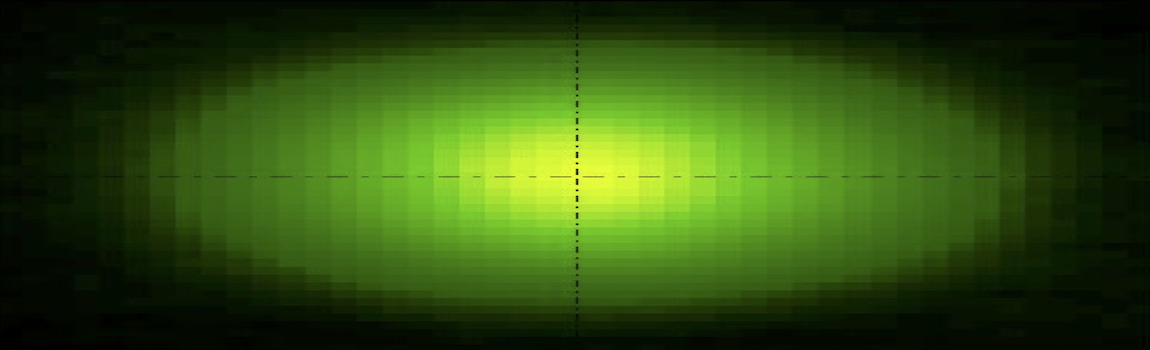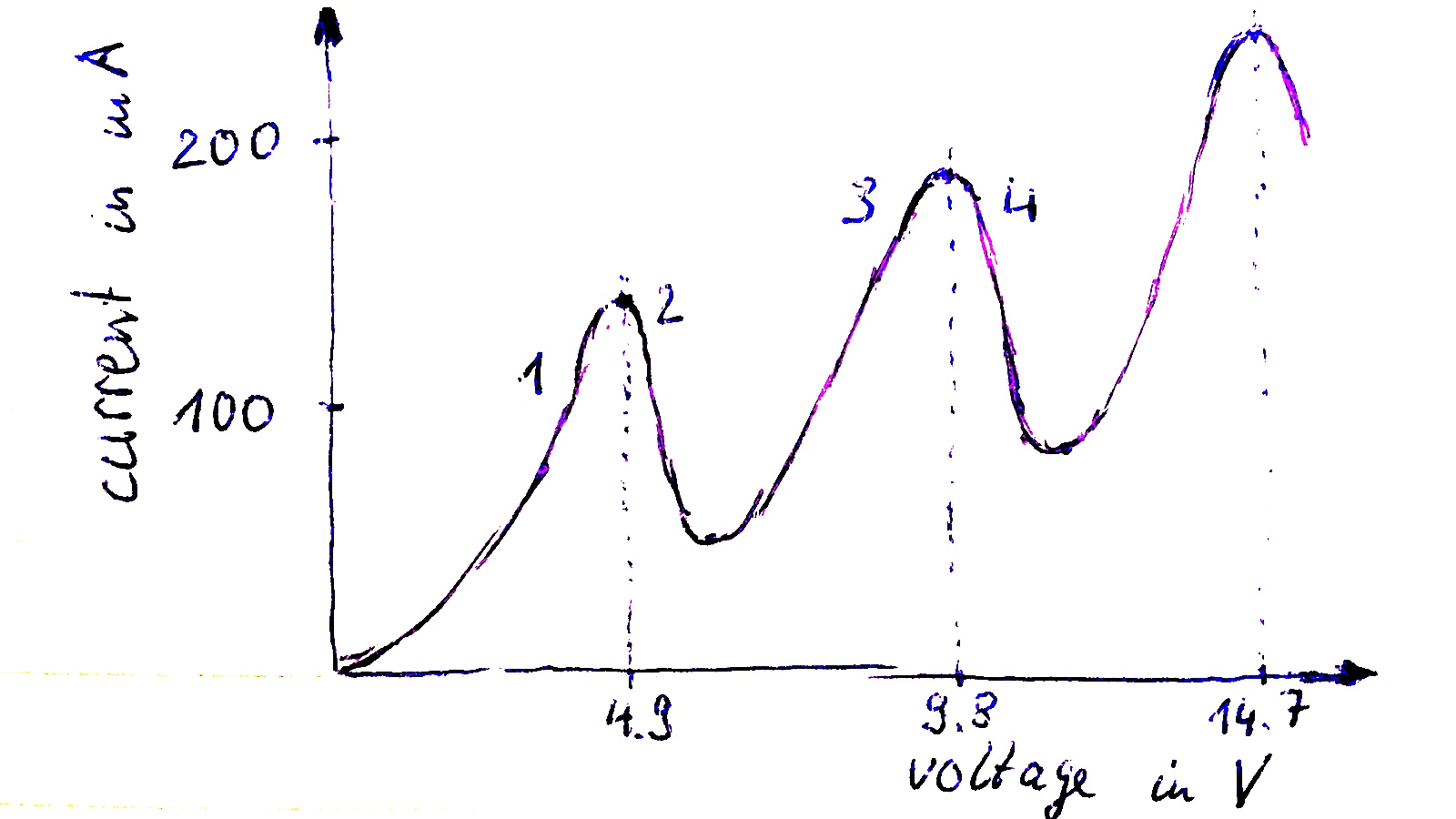# Franck Hertz Experiment

### Observe the experiment

1925 Franck Hertz got the nobel prize in physics for verifying the exis­tence of discrete energy levels in atoms.

He used a tube filled with vaporized mercury vapor. Electrons were accelerated after thermionic emission in the vapor and when he turned up the acceleration voltage suddenly the vapor started a glowing on one specific area close to the anode. When he turned up further the area moved towards the cathode and suddenly there was a second glowing area. So he could produce several glowing areas.

### The experimental setup

For further investigation he chose the following setup: The anode he made out of a metal mesh grid so accelerated electrons could even pass and reach another cathode with a counter voltage of just 1 V.

When he turned up the acceleration voltage he could detect an increasing current at the second cathode.### Results of the experiment1 The current increases until the first area of glowing arises. While the electrons gain more and more speed they will pass through the metal mesh grid and reach the cathode.

2 When the first area of glowing arises the current drops suddenly, since the electrons lose their kinetic energy by inelastic collision with the mercury atoms. The absorbed energy is observed by spontaneous emission of light. The electrons reaches the terminal velocity of $$v=\sqrt{\frac{2eV}{m_e}}$$

3 By further turning up the voltage, the electrons gain again more energy. So the current increases again until the next glowing.

4 The accelerating voltage reaches the double of 4.9 V, so the electrons have again enough energy for inelastic collision producing the next glowing area.

### Questions

The Franck-Hertz experiment verifies the existence of discrete energy levels in atoms.

1. Sketch the experimental setup for the Franck-Hertz experiment.
2. Name the three voltages which are necessary for the experiment.
3. The graph was obtained using a tube containing neon. Explain the physical processes which are responsible for the shape of the voltage-current-graph.
4. Calculate the wavelength and frequency of the emitted radiation.
5. Explain the process where the radiation comes from.
6. Decide whether or not the emitted radiation is within the visible spectrum.### Terms and phrases

 Franck Hertz experiment The Franck-Hertz experiment verifies the existence of discrete energy levels in atoms. heating voltage $V_h$ voltage for thermionic emission accelerating voltage $V_{acc}$ voltage for accelerating liberated electrons counter voltage $V_{counter}$ only electrons which have enough kinetic energy to overcome the counter voltage will be detected.
 inelastic collision if the electrons have enough kinetic energy they cause inelastic collision, causing the atom to an excited state spontaneous emission when the excited atoms come back to the unexcited state, they get rid of the energy by spontaneous emission of radiation. terminal velocity $v$ of electrons before inelastic collision, electrons reach their terminal velocity: $v=\sqrt{\frac{2eV}{m_e}}$